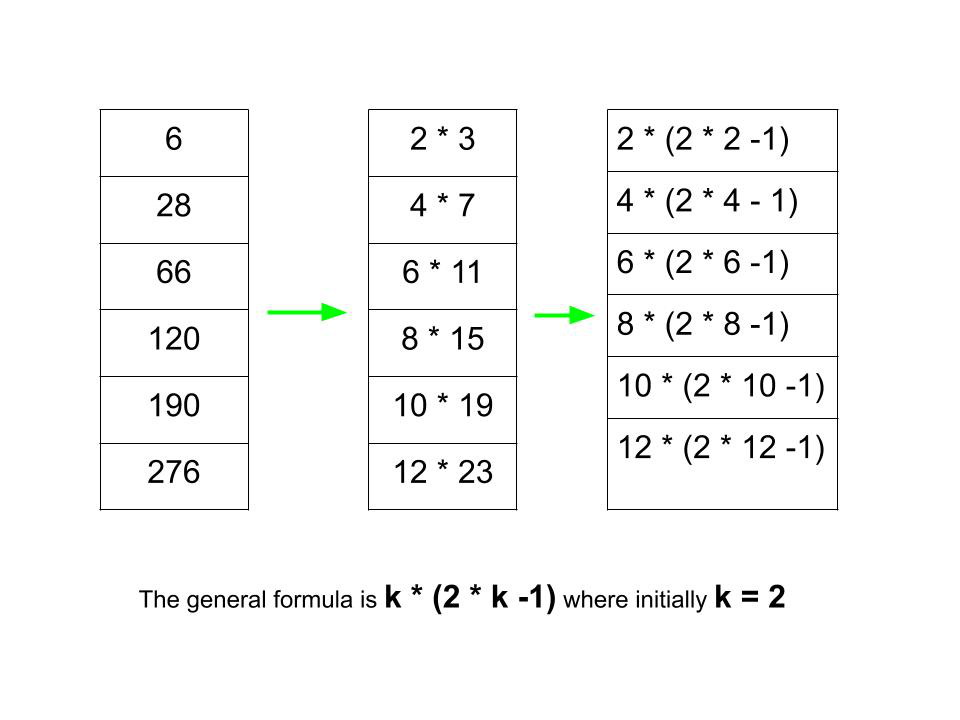GeeksforGeeks App
Open AppBrowser
Continue

# Print the first N terms of the series 6, 28, 66, 120, 190, 276, …

Given a number N, the task is to print the first N terms of the series 6, 28, 66, 120, 190, 276, and so on.
Examples:

Input: N = 10
Output: 6 28 66 120 190 276 378 496 630 780
Input: N = 4
Output: 6 28 66 120

Approach: To solve the problem mentioned above, we have to observe the below pattern:The general formula is given by:
k * (2 * k – 1), where initially, k = 2

Below is the implementation of the above approach:

## C++

 `// C++ program for the above approach``#include ``using` `namespace` `std;` `// Function to print the series``void` `printSeries(``int` `n)``{``    ``// Initialise the value of k with 2``    ``int` `k = 2;` `    ``// Iterate from 1 to n``    ``for` `(``int` `i = 0; i < n; i++) {` `        ``// Print each number``        ``cout << (k * (2 * k - 1))``             ``<< ``" "``;` `        ``// Increment the value of``        ``// K by 2 for next number``        ``k += 2;``    ``}` `    ``cout << endl;``}` `// Driver Code``int` `main()``{``    ``// Given number N``    ``int` `N = 12;` `    ``// Function Call``    ``printSeries(N);``    ``return` `0;``}`

## Java

 `// Java program for the above approach``class` `GFG{` `// Function to print the series``static` `void` `printSeries(``int` `n)``{``    ``// Initialise the value of k with 2``    ``int` `k = ``2``;` `    ``// Iterate from 1 to n``    ``for` `(``int` `i = ``0``; i < n; i++)``    ``{` `        ``// Print each number``        ``System.out.print(k * (``2` `* k - ``1``) + ``" "``);` `        ``// Increment the value of``        ``// K by 2 for next number``        ``k += ``2``;``    ``}` `    ``System.out.println();``}` `// Driver code``public` `static` `void` `main(String args[])``{``    ``// Given number N``    ``int` `N = ``12``;` `    ``// Function Call``    ``printSeries(N);``}``}` `// This code is contributed by shivaniisnghss2110`

## Python3

 `# Python3 program for the above approach` `# Function to print the series``def` `PrintSeries(n):``    ` `    ``# Initialise the value of k with 2``    ``k ``=` `2``    ` `    ``# Iterate from 1 to n``    ``for` `i ``in` `range``(``0``, n):``        ` `        ``# Print each number``        ``print``(k ``*` `(``2` `*` `k ``-` `1``), end ``=` `' '``)``        ` `        ``# Increment the value of``        ``# K by 2 for next number``        ``k ``=` `k ``+` `2``        ` `# Driver code    ` `# Given number``n ``=` `12` `# Function Call``PrintSeries(n)` `# This code is contributed by poulami21ghosh  `

## C#

 `// C# program for the above approach``using` `System;` `class` `GFG{` `// Function to print the series``static` `void` `printSeries(``int` `n)``{``    ` `    ``// Initialise the value of k with 2``    ``int` `k = 2;` `    ``// Iterate from 1 to n``    ``for``(``int` `i = 0; i < n; i++)``    ``{` `        ``// Print each number``        ``Console.Write(k * (2 * k - 1) + ``" "``);` `        ``// Increment the value of``        ``// K by 2 for next number``        ``k += 2;``    ``}``    ``Console.WriteLine();``}` `// Driver code``public` `static` `void` `Main()``{``    ` `    ``// Given number N``    ``int` `N = 12;` `    ``// Function call``    ``printSeries(N);``}``}` `// This code is contributed by sanjoy_62`

## Javascript

 ``

Output:

`6 28 66 120 190 276 378 496 630 780 946 1128`

Time Complexity: O(N)
Auxiliary Space: O(1)

My Personal Notes arrow_drop_up